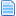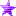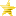# Universal/Anime Trash Buffer System (Read 1466 times)

Started by Websta, September 23, 2019, 12:55:28 pm

#### Websta##### Universal/Anime Trash Buffer System
#1  September 23, 2019, 12:55:28 pm
This is the buffer system I've been using for years now, might as well explain how the thing works.

;=================================

Put this in the Statedef -3

Code:
`[state -3]type = Helpertrigger1 = numhelper(9000) = 0name = "Buffer System"id = 9000pos = 0,0postype = p1stateno = 9000helpertype = normalkeyctrl = 1pausemovetime = 9999999supermovetime = 9999999`

The reason keyctrl is there is because this buffer does motions independently of the root.

Now for the buffer system

Code:
`;---------------------------------------------------------------------------;BUFFER SYSTEM[Statedef 9000]type    = Amovetype= Iphysics = Nctrl = 0anim = 1;  <- THIS IS A BLANK ANIMATION[State 0]; <- MAKES SURE THE BUFFER SYSTEM IS ALWAYS FACING THE OPPONENTtype = turntrigger1 = p2dist x < 0[State 0]type = bindtoroottrigger1 = 1pos = 0,0[State -2]trigger1 = 1type = DisplayToClipboardignorehitpause = 1text = "ID = %d Time = %d rootID = %d"params = var(1), var(2), root,var(2);-------------[State 0]; <- BUFFER TIME FOR MOVES, THIS VARIABLE SET SAYS HOW LONG A MOVE STAYS IN THE BUFFERtype = varsettrigger1 = command = "x"trigger2 = command = "y"trigger3 = command = "z"trigger4 = command = "a"trigger5 = command = "b"trigger6 = command = "c"trigger7 = command = "up"; <- THIS IS FOR BUFFERING JUMPStrigger8 = command = "upfwd";  <-trigger9 = command = "upback"; <-;trigger10 = command = "x+y";trigger11 = command = "x+y+z+a"var(2) = 15:   <- THIS TIMING CAN BE CHANGED TO MAKE THINGS MORE LENIENT OR MORE STRICT[State 0]; <- THE TIMING HERE IS SHORTER BECAUSE THIS IS FOR RUNNING AND BACKDASHINGtype = varsettrigger1 = command = "fwd"trigger2 = command = "back"trigger3 = command = "down"var(2) = 5[State 0]type = varsettriggerall = root,animelemtime(1) = 0trigger1 = root,stateno = [130,139]var(2) = 2[State 0]; <- THIS MAKES SURE THE THE BUFFER TIMER RUNS DOWNtype = varSettrigger1 = var(2)>0var(2) = var(2)-1[State 0]; <- THIS KILLS THE BUFFER TIME WHEN THE MOVE STARTStype = varsettrigger1 = root,animelemtime(1) = 0trigger1 = root,stateno = [200,2999]; <- INCREASE THIS RANGE IF YOUR MOVES USE STATES 3000+var(2) = 0;----[State 0]; <- THIS RESETS THE ID THATS BEING BUFFERED WHEN THE TIMER RUNS OUTtype = varsettrigger1 = var(2) = 0var(1) = 0[State 0] ; <- THIS RESETS THE TIMER WHEN NEUTRAL/STARTING TO JUMP/LANDINGtype = parentvarsettrigger1 = var(2) = 0trigger2 = root,stateno != 40trigger3 = root,stateno != 0trigger4 = root,stateno != 52var(2) = 0;---------------------------;--------------------;NORMAL MOVES;--------------------[State 0]type = varsettriggerall = command != "QCF_x";  <- YOU DONT WANT THIS BUFFERED DOING THIS MOTIONtrigger1 = command = "x"var(1) = 1;  <- THIS IS THE ID OF THE MOVE BUFFERING[State 0]type = varsettriggerall = command != "QCF_y"trigger1 = command = "y"var(1) = 2[State 0]type = varsettriggerall = command != "QCFQCF_z"trigger1 = command = "z"var(1) = 3;--------------------;SPECIAL MOVES;--------------------[State 0];  <- JUST GIVING MOTIONS IDstype = varsettrigger1 = command = "QCF_x"var(1) = 30;  <- THIS IS THE ID OF THE MOVE BUFFERING[State 0]type = varsettrigger1 = command = "QCF_y"var(1) = 31[State 0]; <- THIS IS A SUPER MOTIONtype = varsettrigger1 = command = "QCFQCF_z"var(1) = 32;--------------------;SYSTEM;--------------------[State 0];  <- BUFFERING JUMPStype = varsettriggerall = var(1) != [60,62]triggerall = root,stateno != 40trigger1 = command = "holdupfwd"var(1) = 60[State 0];  <- BUFFERING JUMPStype = varsettriggerall = var(1) != [60,62]triggerall = root,stateno != 40trigger1 = command = "holdupback"var(1) = 61[State 0];  <- BUFFERING JUMPStype = varsettriggerall = var(1) != [60,62]triggerall = root,stateno != 40triggerall = command != "holdupfwd"triggerall = command != "holdupback"trigger1 = command = "holdup"var(1) = 62[State 0];  <- BUFFERING RUNtype = varsettrigger1 = command = "FF"var(1) = 50[State 0];  <- BUFFERING BACKDASHtype = varsettrigger1 = command = "BB"var(1) = 51;---------------------------[State 0];  <- IF THERES NOTHING BEING BUFFERED, THE BUFFER TIMER DIEStype = parentvarsettrigger1 = var(1) = 0var(2) = 0;---------------------------;FROM THIS SECTION ONWARDS ALL THIS TELLS THE ROOT WHAT CONDITIONS ARE REQUIRED FOR A MOVE HAPPEN;---------------------------;==========================;NORMALS;==========================[State 0]type = parentvarsettriggerall = var(1) = 1 && var(2) != 0: <- WHEN THIS ID IS ACTIVE, AND TIMER HAS NO RUN OUT YETtriggerall = root,statetype != A && root,command != "holddown"trigger1 = (root,stateno = [0,52]) && root,ctrl = 1;  <- CONDITIONS FOR WHEN ITS OKAY FOR THIS MOVE TO HAPPENtrigger2 = root,stateno = [100,101]: <-;----var(2) = 1[State 0]type = parentvarsettriggerall = var(1) = 2 && var(2) != 0triggerall = root,statetype != A && root,command != "holddown"trigger1 = (root,stateno = [0,52]) && root,ctrl = 1trigger2 = root,stateno = [100,101];----var(2) = 2[State 0]type = parentvarsettriggerall = var(1) = 3 && var(2) != 0triggerall = root,statetype != A && root,command != "holddown"trigger1 = (root,stateno = [0,52]) && root,ctrl = 1trigger2 = root,stateno = [100,101];----var(2) = 3;==========================;SPECIAL MOVES;==========================[State 0, QCF_x]; <- EVERYTHING IS THE PRETTY MUCH THE SAME, JUST ADD WHAT CONDITIONS YOU WANT FOR THE MOVE TO WORKtype = parentvarsettriggerall = var(1) = 30 && var(2) != 0triggerall = root,statetype != Atrigger1 = (root,stateno = [0,52]) && root,ctrl = 1trigger2 = root,stateno = [100,101];----trigger3 = root,stateno = 200 && root,movecontact && root,numtarget:  <- BASIC NORMAL>SPECIAL CANCEL ON CONTACTtrigger4 = root,stateno = 210 && root,movecontact && root,numtarget;  <-trigger5 = root,stateno = 220 && root,movecontact && root,numtarget:  <-;----var(2) = 30[State 0, QCF_y]type = parentvarsettriggerall = var(1) = 31 && var(2) != 0triggerall = root,statetype != Atrigger1 = (root,stateno = [0,52]) && root,ctrl = 1trigger2 = root,stateno = [100,101];----trigger3 = root,stateno = 200 && root,movecontact && root,numtargettrigger4 = root,stateno = 210 && root,movecontact && root,numtargettrigger5 = root,stateno = 220 && root,movecontact && root,numtarget;----var(2) = 31[State 0, QCFQCF_z]type = parentvarsettriggerall = var(1) = 32 && var(2) != 0triggerall = power >= 1000; <- POWER REQUIREMENT BECAUSE ITS A SUPERtriggerall = root,statetype != Atrigger1 = (root,stateno = [0,52]) && root,ctrl = 1trigger2 = root,stateno = [100,101];----trigger3 = root,stateno = 200 && root,movecontact && root,numtargettrigger4 = root,stateno = 210 && root,movecontact && root,numtargettrigger5 = root,stateno = 220 && root,movecontact && root,numtargetvar(2) = 32;==========================;SYSTEM;==========================[State 0];  <- JUMP CANCELING (YOU MIGHT WANT TO HAVE A LIMITER FOR THIS)type = parentvarsettriggerall = var(1) = 60 && var(2) != 0trigger1 = root,movecontact && root,numtargettrigger1 = root,stateno = [200,499]var(2) = 60[State 0]type = parentvarsettriggerall = var(1) = 61 && var(2) != 0trigger1 = root,movecontact && root,numtargettrigger1 = root,stateno = [200,499]var(2) = 61[State 0]type = parentvarsettriggerall = var(1) = 62 && var(2) != 0 trigger1 = root,movecontact && root,numtargettrigger1 = root,stateno = [200,499]var(2) = 62[State 0];  <- RUN BUFFERtype = parentvarsettriggerall = var(1) = 50 && var(2) != 0triggerall = root,statetype != Atrigger1 = (root,stateno = [0,52]) && root,ctrl = 1var(2) = 50[State 0];  <- BACKDASH BUFFERtype = parentvarsettriggerall = var(1) = 51 && var(2) != 0triggerall = root,statetype != Atrigger1 = (root,stateno = [0,52]) && root,ctrl = 1var(2) = 51`

The buffer system handles all the timing and commands, and when you conditions are met will tell the route what move ID to use.

Add this to the Statedef -2

Code:
`;================================================================================; ------- BUFFER SYSTEM--------;================================================================================[State -3]: <- IF YOU GET HIT, BUFFER SYSTEM SWITCHES OFFtype = varsettriggerall = var(2) != 60triggerall = stateno != [120,159]trigger1 = movetype = Hvar(2) = 0ignorehitpause = 0;==========================;NORMALS;==========================[State -3]; <- MAKES CHANGE TO THE STATE BASED ON THE BUFFER SYSTEMtype = changestatetriggerall = movetype != Htriggerall = roundstate = 2trigger1 = var(2) = 1value = 200ctrl = 0ignorehitpause = 0[State -3]type = changestatetriggerall = movetype != Htriggerall = roundstate = 2trigger1 = var(2) = 2value = 210ctrl = 0ignorehitpause = 0[State -3]type = changestatetriggerall = movetype != Htriggerall = roundstate = 2trigger1 = var(2) = 3value = 220ctrl = 0ignorehitpause = 0;==========================;SPECIAL MOVES;==========================[State -3]type = changestatetriggerall = movetype != Htriggerall = roundstate = 2trigger1 = var(2) = 30value = 1000ctrl = 0ignorehitpause = 0[State -3]type = changestatetriggerall = movetype != Htriggerall = roundstate = 2trigger1 = var(2) = 31value = 1001ctrl = 0ignorehitpause = 0[State -3]type = changestatetriggerall = movetype != Htriggerall = roundstate = 2trigger1 = var(2) = 32value = 1010ctrl = 0ignorehitpause = 0;==========================;SYSTEM;==========================[State -3]; <- JUMP CANCELINGtype = changestatetriggerall = movetype != Htriggerall = statetype != Atriggerall = roundstate = 2triggerall = stateno != 40trigger1 = var(2) = 60trigger2 = var(2) = 61trigger3 = var(2) = 62value = 40ctrl = 0ignorehitpause = 0[State -3]; <- RUN BUFFERtype = changestatetriggerall = movetype != Htriggerall = roundstate = 2trigger1 = var(2) = 50value = 100ctrl = 0ignorehitpause = 0[State -3]; <- BACKDASH BUFFERtype = changestatetriggerall = movetype != Htriggerall = statetype != Atriggerall = roundstate = 2trigger1 = var(2) = 51value = 105ctrl = 0ignorehitpause = 0;================================================================================`

This section causes to root to change to the correct state based on the ID that was buffered

;=================================================================================

The commands used here look like this:

Code:
`[Command]name = "QCF_x"command = ~D, DF, F, x[Command]name = "QCF_y"command = ~D, DF, F, y[Command]name = "QCFQCF_z"command = ~D, DF, F, D, DF, z `

the .cmd times
Code:
`command.time = 15command.buffer.time = 1`

;===============================================

i know some stuff might not be super clear (never made a tutorial thing before)
If you need any explanations feel free to drop a comment---
Last Edit: September 23, 2019, 04:34:12 pm by Websta

#### Mr. Giang##### Re: Universal/Anime Trash Buffer System
#2  September 23, 2019, 01:19:46 pm
••• The Illusionary Wanderer
• I have a dream
Just a small feedback thingy: I think it is better to put the block of codes for Normal moves below SPECIAL MOVES. We know that MUGEN reads everything from above to below, which is, I assume, why you put "triggerall = command != "QCF_x"" in the buffer motion for a single button press (for normal attacks). By putting the said block of codes below SPECIAL MOVES, this will eliminate the need of writing extra line of codes, which will help in a long run (or I might miss something pretty important here).
Also, I think it will help if we have some examples for what kind of commands should we put in -1 and what could be the ideal command.time and command.buffer.time.
Otherwise, tis a pretty insightful thread! Thanks for sharing!

#### Websta##### Re: Universal/Anime Trash Buffer System
#3  September 23, 2019, 01:24:48 pm
By putting the said block of codes below SPECIAL MOVES, this will eliminate the need of writing extra line of codes, which will help in a long run (or I might miss something pretty important here).

Yea, you probably right, not sure why i didnt do it :/
disregard that, commands dont work without if you swap 'em for some reason

Also, I think it will help if we have some examples for what kind of commands should we put in -1 and what could be the ideal command.time and command.buffer.time.
Otherwise, tis a pretty insightful thread! Thanks for sharing!

the commands that you use can be any you feel comfortable with. and the command.time/command.buffer.time i left that at default (15/1)
i'll add the commands used to the top tho---
Last Edit: September 23, 2019, 01:35:12 pm by Websta

#### Nep Heart##### Re: Universal/Anime Trash Buffer System
#4  September 23, 2019, 03:31:11 pm
A small flaw that I'd like to point out is that resetting the buffer whenever the root is in movetype = H would prevent moves that only work while guarding from working since guard hitstates are also movetype = H. Adding an extra trigger like root,stateno != [120,155] should fix that easily.

#### Websta##### Re: Universal/Anime Trash Buffer System
#5  September 23, 2019, 04:07:53 pm
you're right, turns out i missed that chunk when i was making the template---

#### Mr. Giang##### Re: Universal/Anime Trash Buffer System
#6  September 25, 2019, 11:32:46 am
••• The Illusionary Wanderer
• I have a dream
Forgot to ask about this at the first place but since your buffer system uses normal commands, how do you deal with charge/360/720/pretzel motion? MUGEN default buffer tends to screw these commands up (at least for keyboard players) and none of your characters have to deal with it so I have no idea what I should do in that case.

#### Websta##### Re: Universal/Anime Trash Buffer System
#7  September 25, 2019, 11:55:47 am
any commands that you'd normally use in your cmds should work